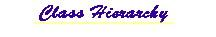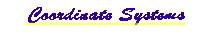## mil.navy.nps.rra Class Lobe

```java.lang.Object
|
+--mil.navy.nps.rra.Lobe
```

public class Lobe
extends java.lang.Object

A Lobe is defined by combining multiple Beams, which in turn are made up of Rays.

Lobes are emitted in a pattern from an source. In a properly designed active sonar, there is only one main Lobe. This class simulates that Lobe. Lobes are characterized by a direction that they are pointed in, and also (typically) by a horizontal and vertical beamwidth. Within this Lobe resides the acoustic energy used for detection of distant objects. This class subdivides the Lobe into Beams. This approach keeps the wave front of each individual bundle of rays from getting significantly larger than the wavelength of the acoustic energy. This constraint also ensures that accuracy is maintained and so many special problems with large area bundle reflection can be ignored. Thus, the Lobe class divides the sonar lobe into a matrix of beams, and divides the energy in the lobe among the beams. Each beam is then calculated individually using the Beam class.

Future Work:
To optimize the execution time, repeated calculation of duplicate rays needs to be eliminated.

Version:
1.0

Author:
LT Timothy M. Holliday USN

Contact: Don Brutzman (web.nps.navy.mil/~brutzman) brutzman@nps.navy.mil

Source Code:
~vrtp/mil/navy/nps/rra/Lobe.java
http://devo.stl.nps.navy.mil/~brutzman/vrtp/mil/navy/nps/rra/Lobe.javaHistory:
 29 Nov 97 Timothy M. Holliday New 17 Mar 98 Timothy M. Holliday Added HTML comment convention 12 Apr 98 Timothy M. Holliday Parameterless Constructors 14 Apr 98 Timothy M. Holliday Simplified VRML Routines 9 Oct 98 Don Brutzman Javadoc updates for jdk1.2b4 25 April 99 Don Brutzman Javadoc updates for jdk1.2.1

Reference:
Holliday, Timothy, Real-Time 3D Sonar Modeling and Visualization, Master's Thesis, Naval Postgraduate School, Monterey California, June 1998. Available at vrtp/rra/HollidayRRAthesis.pdf. and devo.stl.nps.navy.mil/~brutzman/vrtp/rra/HollidayRRAthesis.pdf. Slideset available in Powerpoint and Acrobat .pdf formats.

`Ray`, `Beam`, `Bottom`, `Surface`, `SSP`, `ExampleLobeStatic`, `ExampleLobeDynamic`

 Constructor Summary `Lobe()`           A Lobe is defined as an (m x n) array of Beams.

 Method Summary ` void` `calculateLobe(Targets targets)`           This method calculates the Lobe by calling the calculateBeam method for each beam in the lobe. ` java.lang.String` `detectionVRML()`           This method returns VRML objects that signify detections that were made in the virtual world. ` java.lang.String` `dynamicVRML()`           This method writes the dynamic VRML representation of the Lobe by calling the VRMLBeam routine for each beam. `static boolean` `getAppendLineFeed()`           This is a static method that returns the current line appendage. ` double` `getAzimuth()`           This method to gets the Azimuthatl Angle. ` Bottom` `getBottom()`           This method returns the handle to the bottom object. ` double` `getDeltaTime()`           This method returns the simulation step time. ` int` `getDuration()`           This is an accessor method. ` double` `getElevation()`           This method returns the elevation angle. ` double` `getEndTime()`           This method returns the simulation end time. ` double` `getLobeWidthX()`           This is an accessor method to get the total lobe width in the azimuthal direction reletive to the Ziomek Coordinate System. ` double` `getLobeWidthY()`           This is an accessor method to get the total lobe width in the elevation direction reletive to the Ziomek Coordinate System. ` int` `getNumberXPartition()`           This method returns the number of beams in the azimuthal direction that there are in the lobe. ` int` `getNumberYPartition()`           This method returns the number of beams in the elevation direction that there are in the lobe. ` Vec3d` `getPosition()`           This method returns the lobe position. ` SSP` `getSsp()`           This method returns the handle to the sound speed profile object. ` Surface` `getSurface()`           This method returns the handle to the surface object. ` void` `reset()`           This method resets all of the lobe parameters after instantiation has occurred since reuse is more time efficient than garbage collection and reallocation. `static void` `setAppendLineFeed(boolean pAppendLineFeed)`           This is a static method used to indicate whether a line feed is desired at the end of every line. ` void` `setAzimuth(double phi)`           This method sets the azimuthal angle, which is the angle from the x-axis to the z-axis rotating about the y-axis. ` void` `setBottom(Bottom pBottom)`           This method sets the handle to the bottom object. ` void` `setDeltaTime(double pDeltaTime)`           This method sets the time step in the simulation. ` void` `setDuration(int duration)`           This is an accessor method. ` void` `setElevation(double beta)`           This method sets the elevation angle, which is the angle from the y-axis to the x-axis rotating about the z-axis . ` void` `setEndTime(double pEndTime)`           This method sets the simulation end time. ` void` `setLobeWidthX(double pLobeWidthX)`           This method to sets the total lobe width in the azimuthal direction reletive to the Ziomek Coordinate System. ` void` `setLobeWidthY(double pLobeWidthY)`           This is an accessor method to set the total lobe width in the elevation direction reletive to the Ziomek Coordinate System. ` void` `setNumberXPartition(int number)`           This method sets the number of beams in the azimuthal direction that there are in the lobe. ` void` `setNumberYPartition(int number)`           This method sets the number of beams in the elevation direction that there are in the lobe. ` void` `setPosition(Vec3d pPosition)`           This method sets the lobe positon. ` void` `setSsp(SSP pSsp)`           This method sets the handle to the sound speed profile object. ` void` `setSurface(Surface pSurface)`           This method sets the handle to the surface object. ` java.lang.String` ```staticVRML(int colorMap, int intensityMap)```           This method writes the static VRML representation of the Lobe by calling the VRMLBeam routine for each beam.

 Methods inherited from class java.lang.Object ```, clone, equals, finalize, getClass, hashCode, notify, notifyAll, toString, wait, wait, wait```

 Constructor Detail

### Lobe

`public Lobe()`
A Lobe is defined as an (m x n) array of Beams. Due to memory and initialization constraints, the current limit on Beams is 5 by 5. Use accessor methods to set initial values of member variables.
 Method Detail

### reset

`public void reset()`
This method resets all of the lobe parameters after instantiation has occurred since reuse is more time efficient than garbage collection and reallocation.

### setAzimuth

`public void setAzimuth(double phi)`
This method sets the azimuthal angle, which is the angle from the x-axis to the z-axis rotating about the y-axis.

### getAzimuth

`public double getAzimuth()`
This method to gets the Azimuthatl Angle.

### setLobeWidthX

`public void setLobeWidthX(double pLobeWidthX)`
This method to sets the total lobe width in the azimuthal direction reletive to the Ziomek Coordinate System. Argument is in degrees.

### getLobeWidthX

`public double getLobeWidthX()`
This is an accessor method to get the total lobe width in the azimuthal direction reletive to the Ziomek Coordinate System. Value is in degrees.

### setElevation

`public void setElevation(double beta)`
This method sets the elevation angle, which is the angle from the y-axis to the x-axis rotating about the z-axis .

### getElevation

`public double getElevation()`
This method returns the elevation angle.

### setLobeWidthY

`public void setLobeWidthY(double pLobeWidthY)`
This is an accessor method to set the total lobe width in the elevation direction reletive to the Ziomek Coordinate System. Argument is in degrees.

### getLobeWidthY

`public double getLobeWidthY()`
This is an accessor method to get the total lobe width in the elevation direction reletive to the Ziomek Coordinate System. Value is in degrees.

### setPosition

`public void setPosition(Vec3d pPosition)`
This method sets the lobe positon.

### getPosition

`public Vec3d getPosition()`
This method returns the lobe position.

### setDeltaTime

`public void setDeltaTime(double pDeltaTime)`
This method sets the time step in the simulation.

### getDeltaTime

`public double getDeltaTime()`
This method returns the simulation step time.

### setNumberXPartition

`public void setNumberXPartition(int number)`
This method sets the number of beams in the azimuthal direction that there are in the lobe.

### getNumberXPartition

`public int getNumberXPartition()`
This method returns the number of beams in the azimuthal direction that there are in the lobe.

### setNumberYPartition

`public void setNumberYPartition(int number)`
This method sets the number of beams in the elevation direction that there are in the lobe.

### getNumberYPartition

`public int getNumberYPartition()`
This method returns the number of beams in the elevation direction that there are in the lobe.

### setEndTime

`public void setEndTime(double pEndTime)`
This method sets the simulation end time. This value is reletive to the start time which is 0.0.

### getEndTime

`public double getEndTime()`
This method returns the simulation end time.

### setDuration

`public void setDuration(int duration)`
This is an accessor method. Duration is currently 1 or 2. The integer refers to the number of deltTime increments.

### getDuration

`public int getDuration()`
This is an accessor method. Duration is currently 1 or 2. The integer refers to the number of deltTime increments.

### setBottom

`public void setBottom(Bottom pBottom)`
This method sets the handle to the bottom object.

### getBottom

`public Bottom getBottom()`
This method returns the handle to the bottom object.

### setSurface

`public void setSurface(Surface pSurface)`
This method sets the handle to the surface object.

### getSurface

`public Surface getSurface()`
This method returns the handle to the surface object.

### setSsp

`public void setSsp(SSP pSsp)`
This method sets the handle to the sound speed profile object.

### getSsp

`public SSP getSsp()`
This method returns the handle to the sound speed profile object.

### calculateLobe

`public void calculateLobe(Targets targets)`
This method calculates the Lobe by calling the calculateBeam method for each beam in the lobe.

### detectionVRML

`public java.lang.String detectionVRML()`
This method returns VRML objects that signify detections that were made in the virtual world. The objects are collections of red spheres indicating a detect.

### dynamicVRML

`public java.lang.String dynamicVRML()`
This method writes the dynamic VRML representation of the Lobe by calling the VRMLBeam routine for each beam. Also Writes out a Viewpoint node.

### staticVRML

```public java.lang.String staticVRML(int colorMap,
int intensityMap)```
This method writes the static VRML representation of the Lobe by calling the VRMLBeam routine for each beam.

### setAppendLineFeed

`public static void setAppendLineFeed(boolean pAppendLineFeed)`
This is a static method used to indicate whether a line feed is desired at the end of every line. 'true' indicates a linefeed is desired and 'false' indicates that a space is desired"

### getAppendLineFeed

`public static boolean getAppendLineFeed()`
This is a static method that returns the current line appendage.